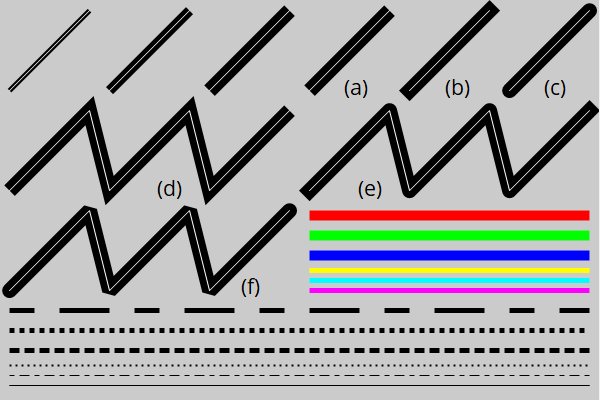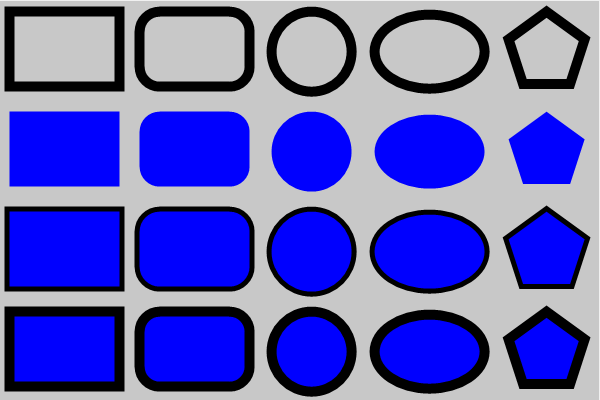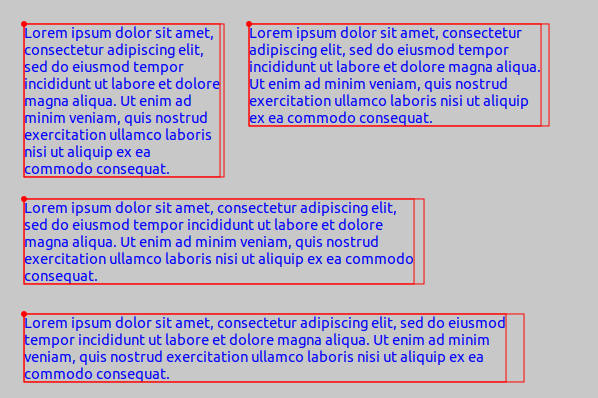# Drawing primitives

This page has been automatically translated using the Google Translate API services. We are working on improving texts. Thank you for your understanding and patience.

## Functions

 void draw_line (...) void draw_polyline (...) void draw_arc (...) void draw_bezier (...) void draw_line_color (...) void draw_line_fill (...) void draw_line_width (...) void draw_line_cap (...) void draw_line_join (...) void draw_line_dash (...) void draw_rect (...) void draw_rndrect (...) void draw_circle (...) void draw_ellipse (...) void draw_polygon (...) void draw_fill_color (...) void draw_fill_linear (...) void draw_fill_matrix (...) void draw_fill_wrap (...) void draw_font (...) void draw_text_color (...) void draw_text (...) void draw_text_path (...) void draw_text_width (...) void draw_text_trim (...) void draw_text_align (...) void draw_text_halign (...) void draw_text_extents (...) void draw_image (...) void draw_image_frame (...) void draw_image_align (...)

When drawing in 2D contexts we have a series of elementary shapes such as lines, figures, text and images. In DrawHello you have the source code of the application that will accompany us throughout this section.

## 1. Line drawing

The most elementary operation is to draw a line between two points. In 2d contexts the lines are solid objects and not a mere row of pixels. Let's think we are using thick tip pens, where the theoretical line will always remain in the center of the stroke (Figure 1). We can change the shape of the endings (linecap), the joints (linejoin) and establish a pattern for dashed lines.

• draw_line will draw a line.
• draw_polyline will draw several connected lines.
• draw_arc will draw an arc.
• draw_bezier will draw a Bézier curve of degree 3 (cubic).
• draw_line_color will set the line color.
• draw_line_width set the line width.
• draw_line_cap set the style of the ends.
• draw_line_join set the style of the unions.
• draw_line_dash set a dot pattern for dashed lines.
•Figure 1: Different line styles. (a) ekLCFLAT. (b) ekLCSQUARE. (c) ekLCROUND. (d) ekLJMITER. (e) ekLJROUND. (f) ekLJBEVEL. The pattern: [5, 5, 10, 5], [1, 1], [2, 1], [1, 2], [5, 5, 10, 5], NULL.

## 2. Figures and borders

To draw figures or closed areas we have several commands. As we see in (Figure 2) we can draw the outline of the figure, its interior or both. For the contour, the established line style will be taken into account as we have seen in the previous section.

• draw_rect for rectangles.
• draw_rndrect for rectangles with rounded edges.
• draw_circle for circles.
• draw_ellipse for ellipses.
• draw_polygon for polygons.
• draw_fill_color set the area fill color.

As we saw in 2D Contexts, the order in which the operations are performed matters. It is not the same to fill and then draw the outline as vice versa. The center of the stroke will coincide with the theoretical contour of the figure.Figure 2: Stroke only ekSTROKE. Fill only ekFILL. First stroke, then fill ekSKFILL. First fill, then stroke ekFILLSK.

Gradients allow regions to be filled using a gradient instead of a solid color (Figure 3). Several base colors and their relative position along a vector are defined (Listing 2). The positions [0, 1] correspond to the extremes and the values within this range to the possible intermediate stops. Each line perpendicular to the vector defines a uniform color that will extend indefinitely until reaching the limits of the figure to be filled.

• Use draw_fill_linear to activate the fill with gradients.

Since the gradient is defined by a vector, it is possible to set a transformation that changes the way it is applied. This matrix is totally independent from the one applied to drawing primitives draw_matrixf, as we saw in Reference systems.

• Use draw_fill_matrix to set the gradient transformation. With this we can get several effects:
• Global gradient: The gradient will be applied globally to the background, and the figures will be cutouts of the same pattern (Figure 4). To do this we will set the identity matrix as a gradient transformation (Listing 3). It is defined by default.
• Local gradient: The vector is transferred to the origin of the figure or to a point in its near surroundings (Figure 5). With this, we will be able to apply the gradient locally and that only affects a specific figure. In (Listing 4) we have slightly varied the transformation to fix the origin in a corner and not in the center of the ellipse. This may vary depending on the desired effect.

In addition to region fill, gradients can also be applied to lines and contours (Figure 6) (Listing 5).

• Use draw_line_fill to draw the lines with the current fill pattern.

As we have said, the color fill will spread evenly and indefinitely along all the lines perpendicular to the vector, but... What happens outside its limits? In (Listing 6) (Figure 7) the gradient has been defined in x=[200, 400], this measure being lower than the figure to be filled:

• Use draw_fill_wrap to define the behavior of the gradient out of bounds.
• ekFCLAMP the end value is used as a constant in the outer area.
• ekFTILE the color pattern is repeated.
• ekFFLIP the pattern is repeated, but reversing the order which prevents the loss of continuity in color.

## 4. Drawing text

Text rendering is the most important part of the user interface. In the old days, small bitmaps were used with the image of each character, but in the early 90's vector fonts based on Bezier curves came into play. The large number of fonts, the immense set of Unicode characters and the possibility of scaling, rotating, or layout the text in paragraphs was a great technical challenge in those years. Fortunately, all this casuistry is largely solved by the native APIs of each operating system, which allows us to provide a simplified interface to add text to our drawings..

To draw single-line texts, we just have to call the function, passing a UTF8 string (Listing 7) (Figure 8). Previously, we can assign the font, color and alignment.

If the string to be displayed has new lines (character '\n') they will be taken into account and the text will be shown in several lines (Listing 8) (Figure 9). We can also obtain the measure in pixels of a block, useful to integrate the text with other primitives.

If the text does not contain new lines, it will be drawn continuously expanding horizontally. This may not be the most appropriate in long paragraphs, so we can set a maximum width, forcing its drawing in several lines (Listing 9) (Figure 10).Figure 10: Text paragraphs with width limit. The maximum and real width obtained with draw_text_extents are shown.

Finally, we can use draw_text_path to treat the text like any other geometric region, highlighting the border or filling with gradients. In this case draw_text_color will have no effect and the values ​​of draw_fill_color, draw_fill_linear and draw_line_color will be used (Listing 10) (Figure 11).

draw_text is much faster than draw_text_path, so we must limit the use of the latter to what is strictly necessary.

## 5. Drawing images

Images generated procedurally or read from disk can be used as a drawing primitive more (Listing 11) (Figure 12). As with text or other figures, the transformation of the context will affect the geometry of the image.

• Use draw_image to draw an image.
• Use draw_image_frame to draw a sequence of an animation.
• Use draw_image_align to set the alignment of the image with respect to the insertion point.

## 6. Default parameters

Each context maintains certain state parameters. At the beginning of the drawing, either by the method `OnDraw` or after creating the context with dctx_bitmap the default values are those shown in (Table 1):

## draw_line ()

Draw a line.

```void
draw_line(DCtx *ctx,
const real32_t x0,
const real32_t y0,
const real32_t x1,
const real32_t y1);
```
 ctx Drawing context. x0 X coordinate of the first point. y0 Y coordinate of the first point. x1 X coordinate of the second point. y1 Y coordinate of the second point.

## draw_polyline ()

Draw several joined lines.

```void
draw_polyline(DCtx *ctx,
const bool_t closed,
const V2Df *points,
const uint32_t n);
```
 ctx Drawing context. closed `TRUE` to join the last point with the first. points Array of points that compose the polyline. n Number of points.

## draw_arc ()

Draw an arc (circle segment).

```void
draw_arc(DCtx *ctx,
const real32_t x,
const real32_t y,
const real32_t start,
const real32_t sweep);
```
 ctx Drawing context. x X coordinate of the arc center. y Y coordinate of the arc center. radius Arc radius. start Initial angle with respect to the vector `X=[1,0] ` in radians. sweep Sweep angle or arc size in radians.

Remarks

Positive angles are those that rotate from vector X to vector Y. See 2D Vectors.

## draw_bezier ()

Draw a cubic Bézier curve (degree 3) using two endpoints (x0,y0)-(x3,y3) and two intermediate control points (x1,y1)-(x2,y2).

```void
draw_bezier(DCtx *ctx,
const real32_t x0,
const real32_t y0,
const real32_t x1,
const real32_t y1,
const real32_t x2,
const real32_t y2,
const real32_t x3,
const real32_t y3);
```
 ctx Drawing context. x0 X coordinate of the starting point. y0 Y coordinate of the starting point. x1 X coordinate of the first intermediate point. y1 Y coordinate of the first intermediate point. x2 X coordinate of the second intermediate point. y2 Y coordinate of the second intermediate point. x3 X coordinate of end point. y3 Y coordinate of the end point.

## draw_line_color ()

Set the color of drawing lines and contours.

```void
draw_line_color(DCtx *ctx,
const color_t color);
```
 ctx Drawing context. color Line color.

## draw_line_fill ()

Sets the current fill pattern for line drawing.

```void
draw_line_fill(DCtx *ctx);
```
 ctx Drawing context.

Remarks

The fill pattern must have been previously set by draw_fill_linear. See Gradients in lines.

## draw_line_width ()

Set the line thickness.

```void
draw_line_width(DCtx *ctx,
const real32_t width);
```
 ctx Drawing context. width Line width.

## draw_line_cap ()

Set the style of the line ends.

```void
draw_line_cap(DCtx *ctx,
const linecap_t cap);
```
 ctx Drawing context. cap Style.

## draw_line_join ()

Set the style of line junctions.

```void
draw_line_join(DCtx *ctx,
const linejoin_t join);
```
 ctx Drawing context. join Union style.

## draw_line_dash ()

Set a pattern for line drawing.

```void
draw_line_dash(DCtx *ctx,
const real32_t *pattern,
const uint32_t n);
```
 ctx Drawing context. pattern Array of values that define the pattern. n Number of values.

Remarks

The first element of `pattern` defines the length of the first stroke and the second of the first hole, so on. Lengths are scaled by line width draw_line_width, that is, a stroke of length 1 will draw a square of side line_width. Lengths of value 2 equal to twice the line thickness, etc. The pattern will scale proportionally when changing the thickness or zooming through transformations.

## draw_rect ()

Draw a rectangle.

```void
draw_rect(DCtx *ctx,
const drawop_t op,
const real32_t x,
const real32_t y,
const real32_t width,
const real32_t height);
```
 ctx Drawing context. op Drawing operation. x X coordinate of the upper left corner of the rectangle. y Y coordinate of the upper left corner of the rectangle. width Rectangle width. height Rectangle height.

Remarks

In Cartesian systems (x,y) indicate the origin of the lower left corner.

## draw_rndrect ()

Draw a rectangle with rounded edges.

```void
draw_rndrect(DCtx *ctx,
const drawop_t op,
const real32_t x,
const real32_t y,
const real32_t width,
const real32_t height,
```
 ctx Drawing context. op Drawing operation. x X coordinate of the upper left corner of the rectangle. y Y coordinate of the upper left corner of the rectangle. width Rectangle width. height Rectangle height. radius Corner curvature radius.

Remarks

In Cartesian systems (x,y) indicate the origin of the lower left corner.

## draw_circle ()

Draw a circle.

```void
draw_circle(DCtx *ctx,
const drawop_t op,
const real32_t x,
const real32_t y,
```
 ctx Drawing context. op Drawing operation. x X coordinate of the center. y Y coordinate of the center. radius Radius.

## draw_ellipse ()

Draw an ellipse.

```void
draw_ellipse(DCtx *ctx,
const drawop_t op,
const real32_t x,
const real32_t y,
```
 ctx Drawing context. op Drawing operation. x X coordinate of the center. y Y coordinate of the center. radx X axis radius. rady Y axis radius.

## draw_polygon ()

Draw a polygon.

```void
draw_polygon(DCtx *ctx,
const drawop_t op,
const V2Df *points,
const uint32_t n);
```
 ctx Drawing context. op Drawing operation. points Array of points that form the polygon. n Number of points.

## draw_fill_color ()

Set a solid color for area filling.

```void
draw_fill_color(DCtx *ctx,
const color_t color);
```
 ctx Drawing context. color Fill color.

## draw_fill_linear ()

Set a gradient for filling areas.

```void
draw_fill_linear(DCtx *ctx,
const color_t *color,
const real32_t *stop,
const uint32_t n,
const real32_t x0,
const real32_t y0,
const real32_t x1,
const real32_t y1);
```
 ctx Drawing context. color Color array. stop Color positions. n Number of positions/colors. x0 X coordinate of the starting point. y0 Y coordinate of the starting point. x1 X coordinate of the end point. y1 Y coordinate of the end point.

Remarks

The positions must go from the value 0 to 1. See Gradients.

## draw_fill_matrix ()

Sets the transformation matrix of the fill pattern.

```void
draw_fill_matrix(DCtx *ctx,
const T2Df *t2d);
```
 ctx Drawing context. t2d Transformation.

Remarks

It will only be effective in non-solid fills. See Gradients.

## draw_fill_wrap ()

Set the behavior of the gradient or fill pattern to the limits.

```void
draw_fill_wrap(DCtx *ctx,
const fillwrap_t wrap);
```
 ctx Drawing context. wrap Behavior at the edge.

Remarks

It will only be effective in non-solid fills. See Gradients.

## draw_font ()

Set the font for text drawing.

```void
draw_font(DCtx *ctx,
const Font *font);
```
 ctx Drawing context. font Fuente tipográfica.

Remarks

Tendrá efecto a partir del siguiente texto dibujado. Ver Typography fonts.

## draw_text_color ()

Sets the text color.

```void
draw_text_color(DCtx *ctx,
const color_t color);
```
 ctx Drawing context. color Color.

## draw_text ()

Draw a block of text.

```void
draw_text(DCtx *ctx,
const char_t *text,
const real32_t x,
const real32_t y);
```
 ctx Drawing context. text UTF8 string, terminated in a null character `'\0'`. x X coordinate on the canvas of the text origin. y Y coordinate on the canvas of the text origin.

Remarks

The text will be drawn with the font and preset style and will be sensitive to the context transformation. See Drawing text.

## draw_text_path ()

Draw a block of text as a geometric area. Similar to draw_text, but allows you to use gradients or draw only the border of the text.

```void
draw_text_path(DCtx *ctx,
const drawop_t op,
const char_t *text,
const real32_t x,
const real32_t y);
```
 ctx Drawing context. op Drawing operation. text UTF8 string, null-terminated `'\0'`. x X coordinate on the canvas of the text origin. y Y coordinate on canvas of text origin.

Remarks

The text will be drawn with the preset font and style (fill and line) and will be context sensitive. See Drawing text.

## draw_text_width ()

Set the maximum width of the text blocks.

```void
draw_text_width(DCtx *ctx,
const real32_t width);
```
 ctx Drawing context. width Maximum width.

Remarks

If the text to draw with draw_text is wider than `width`, it will fragment into several lines. Pass -1 to draw the entire block on a single line. Context scaling is not taken into account. The measurement is made based on the size of the preset font. See Drawing text.

## draw_text_trim ()

Sets how the text will be trimmed when it is wider than the value of draw_text_width.

```void
draw_text_trim(DCtx *ctx,
const ellipsis_t ellipsis);
```
 ctx Drawing context. ellipsis Trim style.

## draw_text_align ()

Sets the alignment of the text with respect to the insertion point.

```void
draw_text_align(DCtx *ctx,
const align_t halign,
const align_t valign);
```
 ctx Drawing context. halign Horizontal alignment. valign Vertical alignment.

Remarks

The insertion point is the coordinate `(x,y)` from draw_text. See Drawing text.

## draw_text_halign ()

Set the internal horizontal alignment of the text, within a multi-line block.

```void
draw_text_halign(DCtx *ctx,
const align_t halign);
```
 ctx Drawing context. halign Horizontal alignment.

Remarks

In single-line texts, it has no effect. See Drawing text.

## draw_text_extents ()

Calculate the size of a block of text.

```void
draw_text_extents(DCtx *ctx,
const char_t *text,
const real32_t refwidth,
real32_t *width,
real32_t *height);
```
 ctx Drawing context. text Text. refwidth Reference width. width Block width. height Block height.

Remarks

If `refwidth` is greater than `0`, `width` will be bounded by this value and `height` will expand to accommodate all the text. Take into account possible new lines `'\n'` from `text`.

## draw_image ()

Draw a image.

```void
draw_image(DCtx *ctx,
const real32_t x,
const real32_t y);
```
 ctx Drawing context. x X coordinate on the canvas of the image origin. y Y coordinate on the canvas of the image origin.

Remarks

The image will be drawn at its natural size and in the indicated position. Use draw_matrixf to perform scaling and rotation. See Drawing images.

## draw_image_frame ()

Like draw_image, but indicating the sequence number of an animation.

```void
draw_image_frame(DCtx *ctx,
const uint32_t frame,
const real32_t x,
const real32_t y);
```
 ctx Drawing context. frame Sequence index (frame) of the animation. x X coordinate on the canvas of the image origin. y Y coordinate on the canvas of the image origin.

Remarks

Only images created from a GIF file support multiple frames (animations). See image_num_frames.

## draw_image_align ()

Sets the alignment of the image with respect to the insertion point.

```void
draw_image_align(DCtx *ctx,
const align_t halign,
const align_t valign);
```
 ctx Drawing context. halign Horizontal alignment. valign Vertical alignment.

Remarks

The insertion point is the coordinate `(x,y)` from draw_image. See Drawing images.